# SAT Math Multiple Choice Question 402: Answer and Explanation

### Test Information

Question: 402

12.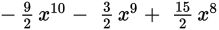Which of the following is equivalent to the expression above?

• A.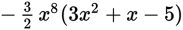• B.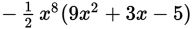• C.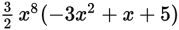• D. 3x8 (-3x2 - x + 5)

Explanation:

A

Difficulty: Medium

Category: Passport to Advanced Math / Exponents

Strategic Advice: Find the greatest common factor (GCF) that can be divided out of all of the terms in the expression. You need to find the greatest number and the variable to the highest power that each term has in common. To make finding the GCF easier, ignore the 2s in the denominators until you've decided on a GCF and then put the 2 back in.

Getting to the Answer: The greatest number that 9, 3, and 15 have in common is 3, so the GCF (with the 2 back in the denominator) is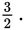All terms in the expression have at least x8, so you can also factor out x8 from each term.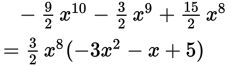Unfortunately, this isn't one of the answer choices. However, in (A),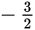has been factored out, and all of the signs of the terms are reversed. This answer is equivalent to the one found above and is therefore correct.